# Same Doubles don't equal

Hi Guys,

Look at this. I have two numbers set as doubles (3731.73 and 3731.74). One is bigger than the other by 0.01

I have two log messages:

First one give me bool result if the two numbers are equal - they are not so I predict to get FALSE as result

Second one give me bool result if the two numbers are equal after I add 0.01 to the lower number to get them the same. I predict to get TRUE as result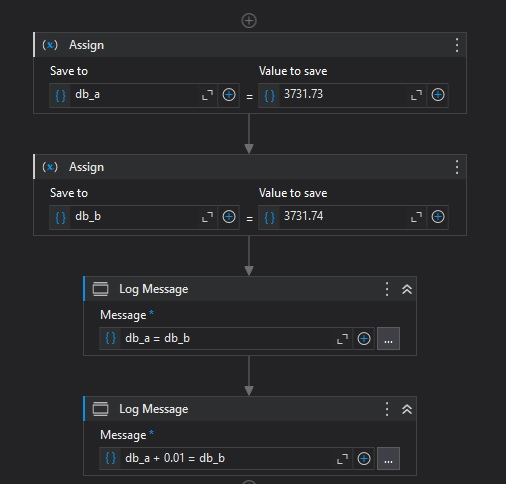When I run the process I get two FALSEs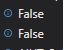What is strange is that if I try any other numbers like 1200.73 and 1200.74 and run the process I get right results ( Which is False and True)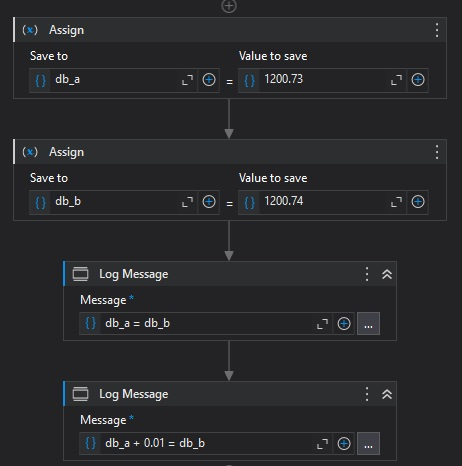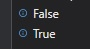What is happening? I tried this on second machine and the results are the same. Youcan try it by yourself. What the heck?

Hi,

Probably it’s double type accuracy matter.
Can you check the following post and check them using `.ToString("G17")`

Regards,

Thanks - your answer is on point. I checked your “G17” idea and I got those results: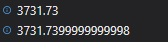I used the “math.Round” when comparing those two numbers (after adding 0.01 to the lower one) and got TRUE as a result which is right.

Thanks for your helpThis topic was automatically closed 3 days after the last reply. New replies are no longer allowed.